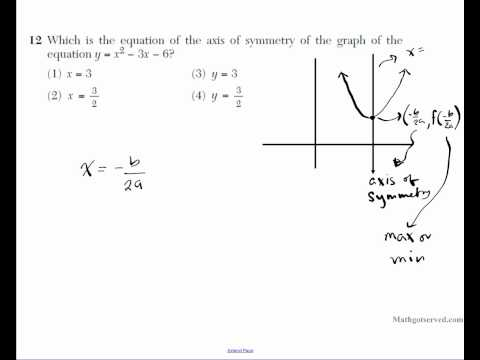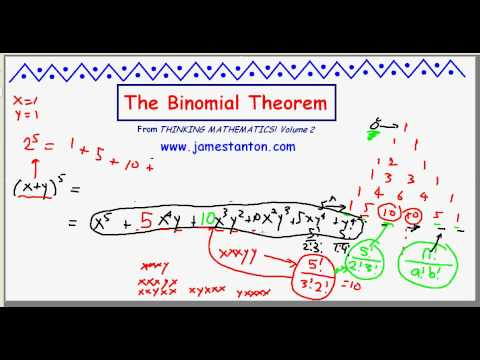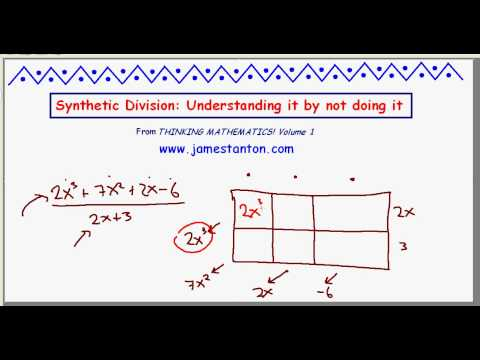## June 2010 NY regents exam Mathematics B part II 11 20 New York State TestFor more cool math videos visit my site at mathgotserved.com or http Algebra Strand Students will represent and analyze algebraically a wide variety of problem solving situations. Variables and AA1 Translate a quantitative verbal phrase into an algebraic Expressions expression AA2 Write a verbal expression that matches a given mathematical expression Equations and AA3 Distinguish the difference between an algebraic Inequalities expression and an algebraic equation AA4 Translate verbal sentences into mathematical equations or inequalities AA5 Write algebraic equations or inequalities that represent a situation AA6 Analyze and solve verbal problems whose solution requires solving a linear equation in one variable or linear inequality in one variable AA7 Analyze and solve verbal problems whose solution requires solving systems of linear equations in two variables AA8 Analyze and solve verbal problems that involve quadratic equations AA9 Analyze and solve verbal problems that involve exponential growth and decay AA10 Solve systems of two linear equations in two variables algebraically (See AG7) AA11 Solve a system of one linear and one quadratic equation in two variables, where only factoring is required Note: The quadratic equation should represent a parabola and the solution(s) should be integers. Students will perform algebraic procedures accurately. Variables and AA12 Multiply and divide monomial expressions with a common Expressions base, using the properties of

## The Binomial Theorem (TANTON Mathematics)Why is expanding a binomial of the form (x + y)^n connected with the n-th row of Pascal’s triangle? (For example, the coefficients that appear when expanding (x + y)^4 are 1, 4, 6, 4,1 and these represent the fourth row of the triangle.) In this video we explain the connection and show how to have fun and prove mysterious properties of the triangle that you can invent for yourself! WARNING: This video does rely on having seen the “A Formula for Pascal’s Triangle” video just so you know what the entries of Pascal’s triangle actually count.

## Synthetic Division: How to understand It by not doing it. (TANTON Mathematics)So many teachers teach their students a completely mysterious and strange algorithm for dividing polynomials by linear terms. Why does it work? What if I want to divide by something other than a linear term. Here I discuss a general method where UNDERSTANDING outweighs and outdoes memorisation!